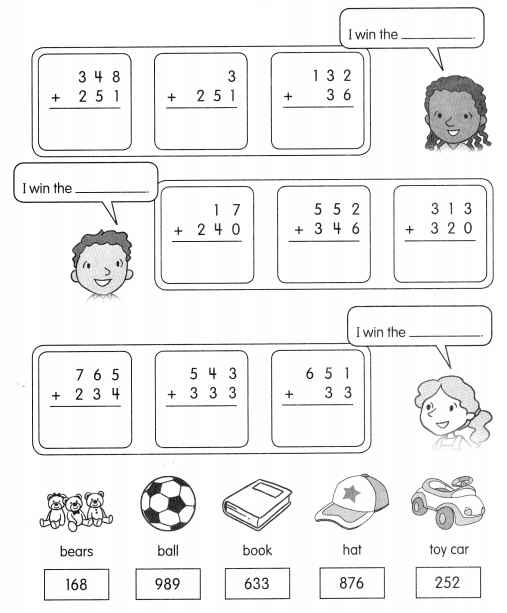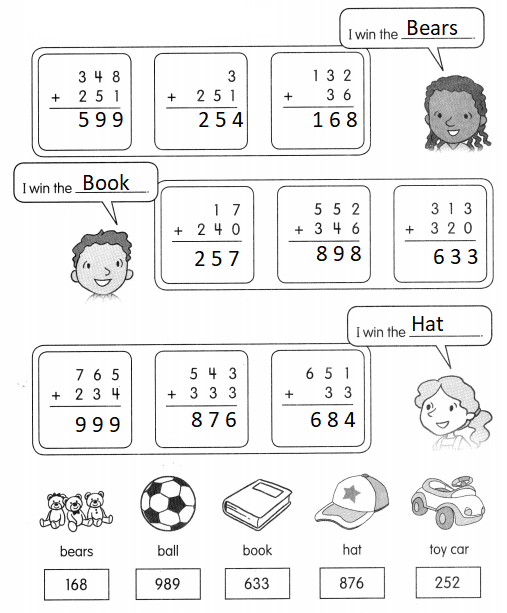Question 1.
232 + 645 = ?
2 ones + 5 ones = 7 ones
3 tens + 4 tens = _________ tens
2 hundreds + 6 hundreds = _______ hundreds
232 ÷ 645 = _______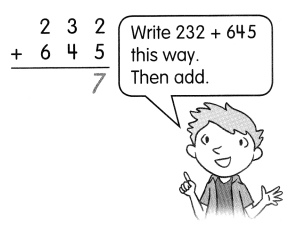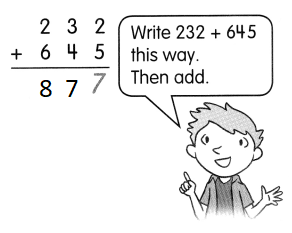2 ones + 5 ones = 7 ones
3 tens + 4 tens = 7 tens
2 hundreds + 6 hundreds = 8 hundreds

Question 2.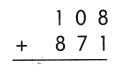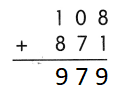Explanation:
8 ones + 1 ones = 9 ones
0 tens + 7 tens = 7 tens
1 hundreds + 8 hundreds = 9 hundreds

Question 3.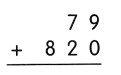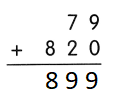Explanation:
9 ones + 0 ones = 9 ones
7 tens + 2 tens = 9 tens
8 hundreds = 8 hundreds

Question 4.
122 + 473 = ___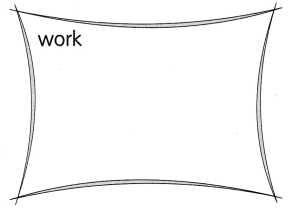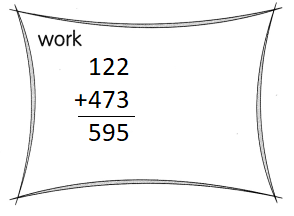Explanation:
The sum of 122 + 473 = 595

Question 5.
217 + 771 = ___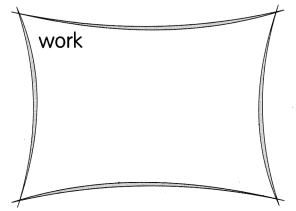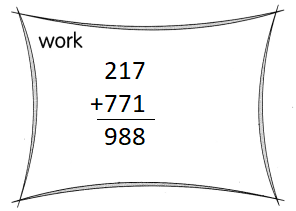Explanation:
The sum of 217 + 771 = 988